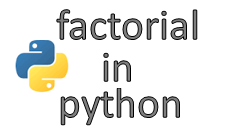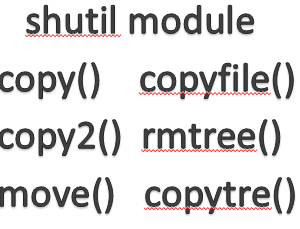## Python Program to Calculate Factorial of a NumberFactorial function is a mathematical formula and used in many areas of mathematics. It is the product of all positive numbers less than or equal to n. Factorial number is represented by an exclamation mark ‘!’.

```1!  = 1 * 1 = 1
3!  = 1 * 2 * 3 = 6
5!  = 1 * 2 * 3 * 4 * 5 = 120
.
.
.
n!  = n * (n-1) * (n-2) * (n-3) . . 2 * 1
```

factorial of  numbers

In Python, factorial number can be found using the different functions as below.

User-defined factorial function.
SciPy factorial function.
Math factorial function.
Numpy factorial function.

All above are the same function of  <function math.factorial> class except first. Check the below screen shot along with it’s output to verify the math class function.

#### User defined factorial function.

Below program demonstrate how to calculate the factorial of  any given number using User defined factorial function .

 ```1 2 3 4 5 6 7``` ```def factorial(user_input): if user_input==0: return 1 elif user_input<0: return 'Factorial does not exist for negative number.' else: return user_input*factorial(user_input-1) ```

scipy.special.factorial(n,exact=True)

```Output:
>>> user_input=int(input('Enter a number :'))
Enter the number :0
>>> print(factorial(user_input))
1

>>> user_input=int(input('Enter a number :'))
Enter the number :-1

>>> print(factorial(user_input))
Factorial does not exist for negative number.>>> user_input=int(input('Enter a number :')) Enter the number :5>>> print(factorial(user_input)) 120```

In above program we takes input from user and store that value to variable (user_input) and pass this value to user defined function(factorial()) to calculate the factorial of given number.

This function checks if the number is 0 it return 1 (0!=1)and if we provides any non positive number to this function then it return output as Factorial does not exist for negative number .Recursive function is used in above example to compute the factorial of given number

#### SciPy factorial function

SciPy is an open-source library for mathematics, science, and engineering. The SciPy library depends on NumPy. Scipy also provides a factorial function to calculate factorial of any number. Scipy provides two different functions to calculate the factorial of the numbers.

scipy.math.factorial() -This function take single parameter (n) and return integer value as output.if n is negatives number then it raise ValueError.

scipy.special.factorial()-This function takes 2 parameters. n-int or array_like of int. exact-If True return integer value and if False it return floating values.By default it return false.

```>>> from scipy import *
>>>scipy.math.factorial(6)
720
>>>scipy.math.factorial(0)
1
>>>scipy.math.factorial(-5)
ValueError: factorial() not defined for negative values
```

scipy.math.factorial(n)

```>>> from scipy import *
>>> scipy.special.factorial(3) #retrun value is integer of nummpy n-dimensional array type
array(6.)

>>> scipy.special.factorial(3,exact=True) #Return value as int if we use exact=True
6>>> scipy.special.factorial(-3) array(0.)
```

scipy.special.factorial(n,exact=True)

#### Math factorial function

Math module in python contains a number of various mathematical operations like sin,sqrt,trunc and log etc. We can also use math module to find factorial of any number using math.factorial() function.

```>>> import math
>>> math.factorial(7)
5040

>>> math.factorial(8)
40320```

scipy.special.factorial(n,exact=True)

#### Numpy factorial function

In numpy ,factorial function take one integer arument to calculate factorial of desired number.if we provide negative argument to this function then python will raise a value error as shown in below example.

```>>> import numpy as np
>>> np.math.factorial(-1)
ValueError: factorial() not defined for negative values

>>> np.math.factorial(4)
24
```

numpy.math.factorial(n)

This is all about factorial number and different functions used to generate the factorial of the desired number. It is not too much complicated to generate factorial of any values using the above mention functions. If you want to see more python function with an interesting example then click here.

Category:Hi, I’m Steven, a Florida native, who left my career in corporate wealth management six years ago to embark on a summer of soul searching that would change the course of my life forever.

• ## The term ‘npm’ is not recognized as the name of a cmdlet, function, script file, or operable program• ## Exploring Essential File Operations in Python with shutil library